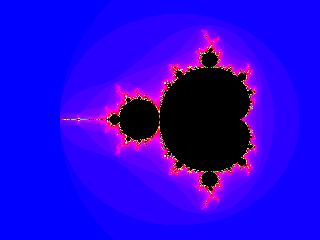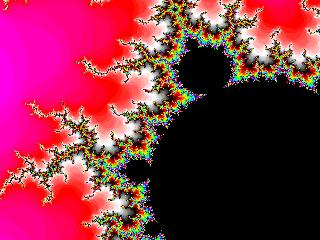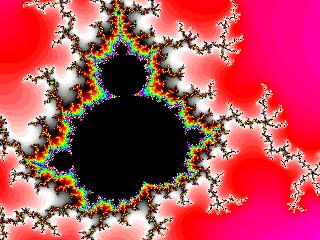## Mandelbrot program

The Mandelbrot set is a fascinating example of a fractal complexity that can be generated from a very simple equation: z = z*z + c. Here is a program to generate an image of the Mandelbrot set:

`/*  A program to generate an image of the Mandelbrot set.   Usage: ./mandelbrot > output         where "output" will be a binary image, 1 byte per pixel         The program will print instructions on stderr as to how to         process the output to produce a JPG file.  Michael Ashley / UNSW / 13-Mar-2003*/// Define the range in x and y here:const double yMin = -1.0;const double yMax = +1.0;const double xMin = -2.0;const double xMax = +0.5;// And here is the resolution:const double dxy = 0.005;#include <stdio.h>#include <limits.h>int main(void) {    double cx, cy;    double zx, zy, new_zx;    unsigned char n;    int nx, ny;    // The Mandelbrot calculation is to iterate the equation    // z = z*z + c, where z and c are complex numbers, z is initially    // zero, and c is the coordinate of the point being tested. If    // the magnitude of z remains less than 2 for ever, then the point    // c is in the Mandelbrot set. We write out the number of iterations    // before the magnitude of z exceeds 2, or UCHAR_MAX, whichever is    // smaller.    for (cy = yMin; cy < yMax; cy += dxy) {        for (cx = xMin; cx < xMax; cx += dxy) {            zx = 0.0;             zy = 0.0;             n = 0;            while ((zx*zx + zy*zy < 4.0) && (n != UCHAR_MAX)) {                new_zx = zx*zx - zy*zy + cx;                zy = 2.0*zx*zy + cy;	        zx = new_zx;	        n++;            }            write (1, &n, sizeof(n)); // Write the result to stdout        }    }    // Now calculate the image dimensions. We use exactly the same    // for loops as above, to guard against any potential rounding errors.    nx = 0;    ny = 0;    for (cx = xMin; cx < xMax; cx += dxy) {        nx++;    }    for (cy = yMin; cy < yMax; cy += dxy) {        ny++;    }    fprintf (stderr, "To process the image: convert -depth 8 -size %dx%d gray:output out.jpg\n",	     nx, ny);    return 0;}`

If you enjoyed that, have a look for the program "fracint" for Windows machines, and "mxp" for GNU/Linux. Here are some examples from "mxp", showing the complete Mandelbrot set first, and then enlargements of various sections of it:The following is an improved version of the above program, using integer arithmetic in the "for" loop, to guarantee the size of the image.

`/*  A program to generate an image of the Mandelbrot set.   Usage: ./mandelbrot > output         where "output" will be a binary image, 1 byte per pixel         The program will print instructions on stderr as to how to         process the output to produce a JPG file.  Michael Ashley / UNSW / 13-Mar-2003*/const double xCentre = -0.75;const double yCentre = +0.0;const int    nx      = 400;const int    ny      = 400;const double dxy     = 0.005;#include <stdio.h>#include <limits.h>int main() {    double cx, cy;    double zx, zy, new_zx;    unsigned char n;    int i, j;    // The Mandelbrot calculation is to iterate the equation    // z = z*z + c, where z and c are complex numbers, z is initially    // zero, and c is the coordinate of the point being tested. If    // the magnitude of z remains less than 2 for ever, then the point    // c is in the Mandelbrot set. We write out the number of iterations    // before the magnitude of z exceeds 2, or UCHAR_MAX, whichever is    // smaller.    for (j = 0; j < ny; j++) {      cy = yCentre + (j - ny/2)*dxy;      for (i = 0; i < nx; i++) {	cx = xCentre + (i - nx/2)*dxy;	zx = 0.0; 	zy = 0.0; 	n = 0;	while ((zx*zx + zy*zy < 4.0) && (n != UCHAR_MAX)) {	  new_zx = zx*zx - zy*zy + cx;	  zy = 2.0*zx*zy + cy;	  zx = new_zx;	  n++;	}	write (1, &n, sizeof(n)); // Write the result to stdout      }    }    fprintf (stderr, "To process the image: convert -depth 8 -size %dx%d gray:output out.jpg\n",	     nx, ny);    return 0;}`
And here is a version using the C-99 complex numbers:
`/*  A program to generate an image of the Mandelbrot set.  Usage: ./mandelbrot > output         where "output" will be a binary image, 1 byte per pixel         The program will print instructions on stderr as to how to         process the output to produce a JPG file.  Michael Ashley / UNSW / 23-Apr-2004*/const double xCentre = -0.75;const double yCentre = +0.0;const int    nx      = 400;const int    ny      = 400;const double dxy     = 0.005;#include <stdio.h>#include <unistd.h>#include <limits.h>#include <complex.h>int main() {    double complex c, z;    unsigned char n;    int i, j;    // The Mandelbrot calculation is to iterate the equation    // z = z*z + c, where z and c are complex numbers, z is initially    // zero, and c is the coordinate of the point being tested. If    // the magnitude of z remains less than 2 for ever, then the point    // c is in the Mandelbrot set. We write out the number of iterations    // before the magnitude of z exceeds 2, or UCHAR_MAX, whichever is    // smaller.    for (j = 0; j < ny; j++) {        for (i = 0; i < nx; i++) {            c = xCentre + (i - nx/2)*dxy +  I * (yCentre + (j - ny/2)*dxy);            z = 0.0;            n = 0;            while ((cabs(z) < 2.0) && (n != UCHAR_MAX)) {                z = z*z + c;                n++;            }            write(1, &n, sizeof(n)); // Write the result to stdout        }    }    fprintf (stderr, "To process the image: convert -depth 8 -size %dx%d gray:output out.jpg\n",         nx, ny);    return 0;}`